# How do you understand these two simple concepts？ (velocity and acceleration)

XinyanWang
Homework Statement:
Is that only need to compare absolute values? And we can ignore the final positive/negative sign, correct？ Why do feel his mind is wrong？
Relevant Equations:
a = Change in velocity / Time taken to change the velocity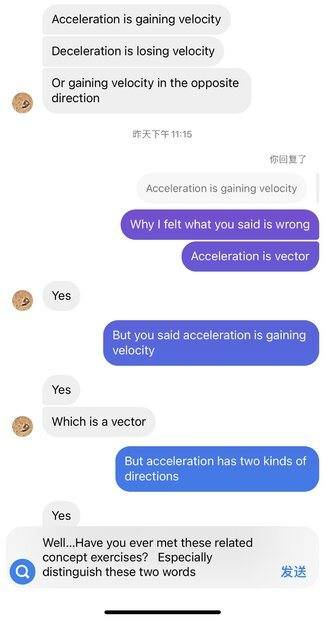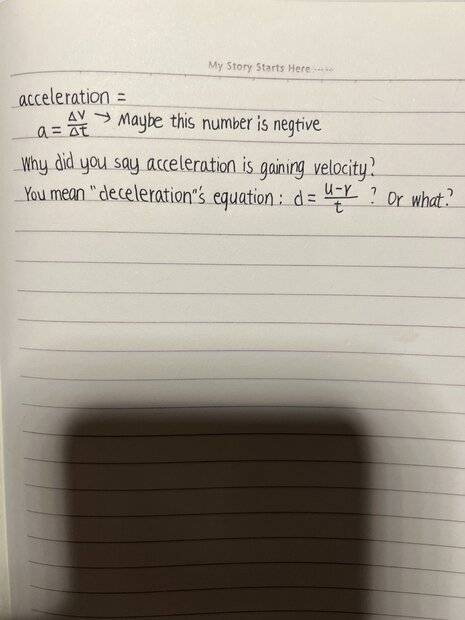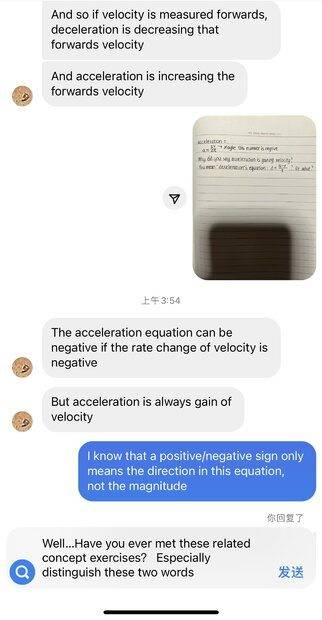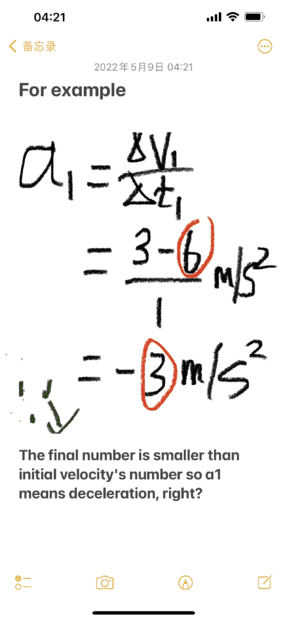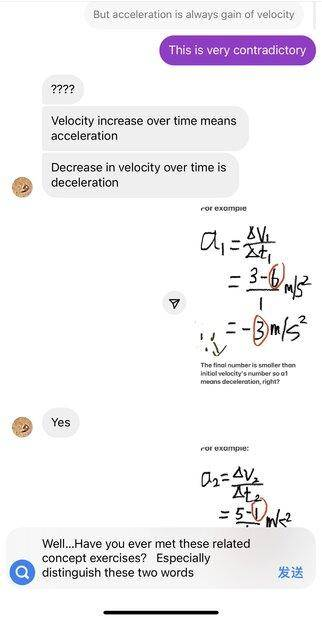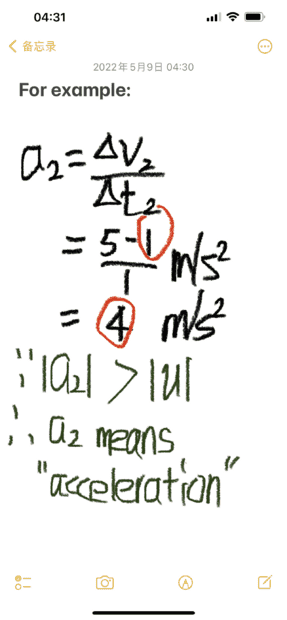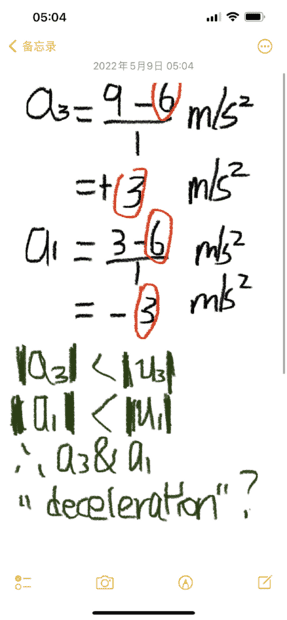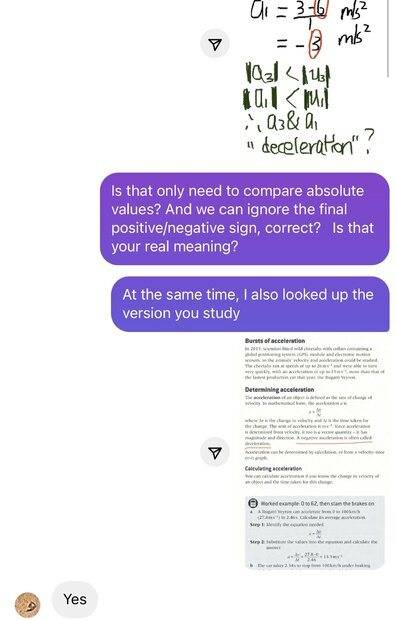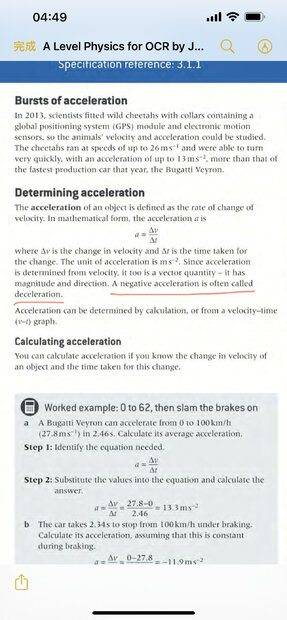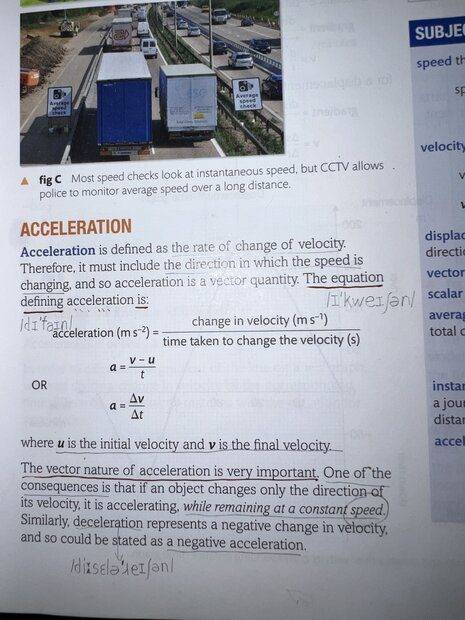Mentor
As the textbook notes, acceleration is the change of velocity. It is a vector, and in one dimension you can reduce it to a scalar which is positive or negative, depending upon the direction.

The use of "deceleration" is every day language, and is seldom used in physics.

In the context of your discussion, it appears that you need to distinguish between acceleration and deceleration. In that case, if ##\vec{a}## is in the same direction as ##\vec{v}##, you have acceleration; if ##\vec{a}## is opposite ##\vec{v}##, you have deceleration. You need to compare the norm of ##\vec{v}## before and after, not of ##\vec{a}##. If ##|\vec{v}_2| < |\vec{v}_1|## (with ##t_2 > t_1##), then the object has decelerated.

•Lnewqban and PeroK
Homework Helper
Gold Member
It might be helpful to distinguish between two kinds of acceleration, the normal (or centripetal) acceleration which changes the direction of velocity and the tangential acceleration which changes the magnitude (or norm) of velocity. Maybe you ll' find this wikipedia article useful.
https://en.wikipedia.org/wiki/Acceleration#Tangential_and_centripetal_acceleration

All I can say is that it seems that your pictured discussion makes a little mess with the terms of acceleration, velocity and speed . It says for example that if velocity increases, then we have acceleration but this is wrong at first place, because velocity is a vector and we can't say that a vector increases. We can say that the magnitude of a vector increases (or decreases). Velocity is a vector, while speed is the magnitude or norm of velocity (lookup magnitude or norm of a vector) and is therefore a scalar. We can say that speed increases or decreases but we just can't say that velocity increases or decreases, UNLESS we have motion in a straight line, where velocity is plus or minus speed depending if the object is moving towards left or right.

Last edited:
•XinyanWang and Lnewqban
Homework Helper
If you want to avoid having people look at you funny, try to avoid phrases such as "accelerate to a stop" in casual English conversation. While technically correct in a physics context, it sounds absolutely wrong in normal speech.

The phrases "speed up" and "slow down" can be used as technically correct and natural replacements for the casual English notions of "accelerate" and "decelerate".

Last edited:
•Delta2, Lnewqban and berkeman
XinyanWang
As the textbook notes, acceleration is the change of velocity. It is a vector, and in one dimension you can reduce it to a scalar which is positive or negative, depending upon the direction.

The use of "deceleration" is every day language, and is seldom used in physics.

In the context of your discussion, it appears that you need to distinguish between acceleration and deceleration. In that case, if ##\vec{a}## is in the same direction as ##\vec{v}##, you have acceleration; if ##\vec{a}## is opposite ##\vec{v}##, you have deceleration. You need to compare the norm of ##\vec{v}## before and after, not of ##\vec{a}##. If ##|\vec{v}_2| < |\vec{v}_1|## (with ##t_2 > t_1##), then the object has decelerated.
I still think your part of understanding is wrong. Because resultant force and mass decide the acceleration. The acceleration's absolute value means how fast this object's velocity changes. It has nothing with velocity and time. When will acceleration appear？ I think this is why the sentence emphasizes again. The vector nature of acceleration is very important. Just like the book says. If an object changes only the direction of velocity, it’s accelerating！！And！"while remaining at a constant speed"！！Constant speed！ Speed doesn’t increase. That's it! A negative change in velocity, that's all! It's like putting a minus sign in front of the delta of velocity change. I feel that the meaning of this word is a pronoun. So could be stated as "a negative acceleration"

Homework Helper
Gold Member
2022 Award
@XinyanWang, please clarify which is your half of the conversation in post #1.

Homework Helper
Gold Member
@XinyanWang also clarify your unorthodox use of exclamation marks. This link explains how and when to use them. They are not meant to be used as separators if that's what you were using them for.

•Delta2
XinyanWang
@XinyanWang, please clarify which is your half of the conversation in post #1.
You mean Instagram conversation？Right

Homework Helper
Gold Member
2022 Award
You mean Instagram conversation？Right
So your text is in the blue/mauve bubbles and your interlocutor's (the other person's) is in the grey bubbles, yes?
Then broadly I agree with you. The other person is confusing magnitude and vector concepts.

It doesn't help that many books get it a bit wrong, describing speed as a scalar. No, scalars are just like 1D vectors, so have a sign. Speed is a magnitude.

In referring to speed in everyday English, an acceleration is an increase in magnitude and deceleration is a reduction in magnitude. In this usage, there is the further ambiguity that acceleration may mean the amount by which the speed increased: "the car accelerated from rest to 30kph", or the rate at which it increased.

But in physics we only use "acceleration" to mean a vector and there is no such concept as deceleration. The extract from "A level physics..." underlined in red, "A negative acceleration is called a deceleration", is nonsense.
And in physics we only use "acceleration" to refer to the rate of change. At least, I would only use it that way, so I have to disagree with this in post @2:
acceleration is the change of velocity

I also object to this statement in the next textbook extract:
"Acceleration is defined as the rate of change of velocity. Therefore it must include the direction in which the speed is changing."
Obviously it should say "the direction in which the velocity is changing."

Even so, there is still an ambiguity. There is average acceleration over an interval of time, ##\vec a_{avg}=\frac{\Delta \vec v}{\Delta t}##, and instantaneous acceleration, the limit of the average acceleration as ##\Delta t## tends to zero. The textbook should make that clearer.

•Lnewqban, Delta2 and jbriggs444
Homework Helper
Gold Member
"A negative acceleration is called a deceleration", is nonsense.
I don't think this statement is nonsense. I think it's incomplete. The completed statement, "If we align the positive axis of coordinates with the velocity, a deceleration is a negative acceleration" makes a lot of sense. The "negative" here is understood as the sign of the 1D scalar component of the acceleration. I hasten to add that I am not advocating the use of "deceleration" in physics because I think it is unnecessary and may lead to negativist confusion, e.g. "Is ##g## positive or negative?" or "Is the amount of heat lost a positive or a negative number?".